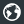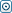Forex Stock Exchange Forum
Would you like to react to this message? Create an account in a few clicks or log in to continue.

# Swapper VertexFX client-side Auto TraderOmSaiTech
Number of messages : 62
Points : 2183
Date of Entry : 2016-05-08
Year : 42
Residence Country : India##Swapper VertexFX client-side Auto Trader

Wed Dec 25, 2019 4:21 am
Swapper Auto Trader is an innovative VertexFX client-side Auto Trader that uses swaps (interest) on open positions to hedge and earn profits.

The concept behind the Swapper Auto Trader is the use of a negative swap (negative interest rate charged on a position) to generate profits. It executes the swap-calculation algorithm at the close of each candle.

In the first step, the Auto Trader calculates the realized profit from all the closed positions. In the next step, the monetary value of the open positions is calculated. Only those positions that have a negative swap are considered in the calculations. The monetary value is computed by multiplying the opening price of each qualifying position with its lot size. The sum of monetary values computed from qualifying long positions is subtracted from the sum of monetary values computed from qualifying short positions to calculate the final (net) fair value.

In the third step, the cumulative lot-size of all positions is calculated. Unlike monetary value, both positive, as well as negative swap positions, are considered. The net cumulative lot-size is the sum of lot sizes of long positions less than the sum of lot sizes of short positions.

In the fourth step, the fair value of the positions in the calculation. The fair value of the positions is the monetary value divided by the cumulative lot-size. It represents the average price at which the positions with negative swap will turn profitable.

If the fair value is greater than zero, the positions are profitable and therefore the latest opened LONG and SHORT position pair is closed. This ensures that the system takes profit whenever it is available and avoids locking in the available margin. Thus the Auto Trader can be efficiently employed on multiple instruments.

If the Auto Trader does not identify any LONG and SHORT position pair to close, it evaluates whether it should open new positions to hedge the negative swap and identify potentially profitable trades. It calculates the price from the highest high of the previous two candles where the profit level can be achieved. Likewise, it calculates the price from the lowest low of the previous two candles where the profit level can be achieved for positions with a negative swap. If the current price is nearer to the upper-profit level, it opens a BUY position. Alternately, if the current price is nearer to the lower profit level, it opens a SHORT position.

It is recommended that the Swapper Auto Trader can be enabled on 15-minute or higher time-frames. Likewise, it is mandatory that the instrument has a positive swap – either the LONG side or the SHORT side should have a positive swap.
Permissions in this forum:
You cannot reply to topics in this forum